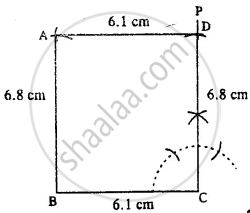# Construct a Rectangle Abcd; If : Bc = 6.1 Cm and Cd = 6.8 Cm. - Mathematics

Sum

Construct a rectangle ABCD; if :

BC = 6.1 cm and CD = 6.8 cm.

#### SolutionSteps:

1. Draw BC = 6.1 cm.
2. At C, draw ∠PCB = 90°.
3. Cut CD = 6.8 cm.
4. Draw an arc of radius 6.8 cm from B.
5. From D, draw an arc of radius 6.1 cm which meets the first arc at A.
Thus ABCD is the required rectangle.
Concept: Construction of a Rectangle When Its Length and Breadth Are Given.
Is there an error in this question or solution?
Chapter 18: Constructions - Exercise 18 (D) [Page 211]

#### APPEARS IN

Selina Concise Mathematics Class 8 ICSE
Chapter 18 Constructions
Exercise 18 (D) | Q 3.2 | Page 211
Share